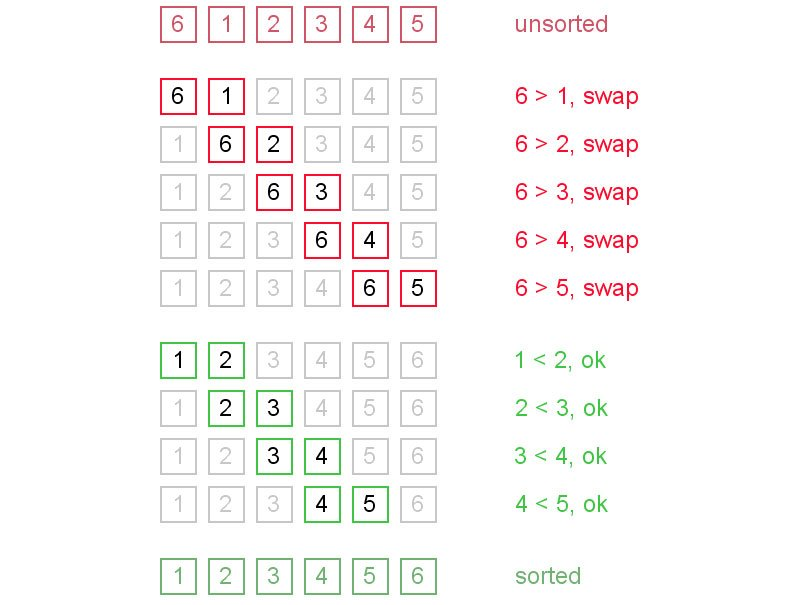## Bubble Sort

Bubble sort, also known as sinking sort, is a simple sorting algorithm that works by repeatedly stepping through the list to be sorted, comparing each pair of adjacent items and swapping them if they are in the wrong order. This process is repeated until no swaps are needed, which indicates that the list is sorted. The algorithm gets its name from the way large elements “bubble” to the bottom of the list (or smaller elements “bubble” to the top of the list depending on your implementation). Because it only uses comparisons to operate on elements, it is sometimes known as a comparison sort. Although the algorithm is simple it is somewhat inefficient for large datasets. In fact, most of the other sorting algorithms are more efficient for larger datasets.## Bubble Sort Complexity

Bubble sort is a quadratic sorting algorithm since it has worst-case and average complexity both О(n2), where n is the number of items being sorted. There exist many sorting algorithms with substantially better worst-case or average complexity of O(n log n). Even other О(n2) sorting algorithms, such as insertion sort, tend to have better performance than bubble sort. Therefore, bubble sort is not a practical sorting algorithm when n is large. Performance of bubble sort over an already-sorted list (best-case) is O(n).

## Bubble Sort Algorithm

Below is an example of Bubble Sort written in Java..

```package miftyisbored;

/**
*
* @author m_yusuf
*	This is a small class that implements Bubble Sort
*/
public class MiftyBubbleSorter{
/**
* This main method is used to illustrate how to use the Bubble Sort Algorithm
**/
public static void main(String[ ] args)
{
final String BLANK_SPACE = "  "; // Just because I am lazy :)
int[ ] data = { 10000, 1002, 83, 12, 58, 72, 66, 65, 75648, 90, 23, 37, 41, 66, 0, 3100, 55555, 1, 67 };

// Print the array before sorting:
System.out.println("Welcome to Mifty's Bubble Sorter");
System.out.println("Here is the original unsorted list:");
for (int i = 0; i < data.length; i++)
System.out.print(data[i] + BLANK_SPACE);
System.out.println( );

// Sort the numbers, and print the results
bubbleSort(data);
System.out.println("All numbers are sorted now!");
System.out.println("Here is the ordered list:");
for (int i = 0; i < data.length; i++)
System.out.print(data[i] + BLANK_SPACE);
System.out.println( );
}

/**
* This function sorts an array of integers from smallest to largest using selection sort
* @param: data - the array to be sorted
*/
public static void bubbleSort(int[ ] data){

int length = data.length;  // get the size of elements
int tempValue; // Used during the swapping of two array values
boolean swapped = true;

while(swapped){
swapped = false;
for (int i = 0; i < length-1; i++) {
if (data[i] > data[i+1]){
// set the swapped flag
swapped = true;

// swap
tempValue = data[i];
data[i] = data[i+1];
data[i+1] = tempValue;
}
}

}

}
}
```

The following is the output of the application

```Welcome to Mifty's Bubble Sorter
Here is the original unsorted list:
10000  1002  83  12  58  72  66  65  75648  90  23  37  41  66  0  3100  55555  1  67
All numbers are sorted now!
Here is the ordered list:
0  1  12  23  37  41  58  65  66  66  67  72  83  90  1002  3100  10000  55555  75648
```

Mifty Yusuf is a Montreal-based software developer who enjoys playing with new web technologies as well as comic books and illustrations. He beleives that, no matter what the question is, the answer is always Batman!